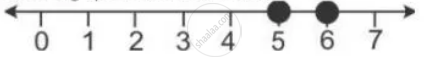Share

# Given that X ∈ I. Solve the Inequation and Graph the Solution on the Number Line: 3 >= (X - 4)/2 + X/3 >= 2 - Mathematics

Course

#### Question

Given that x ∈ I. solve the inequation and graph the solution on the number line:

3 >= (x - 4)/2 + x/3 >= 2

#### Solution

3 >= (x - 4)/2 + x/2 >= 2

3 >= (3x  - 12 + 2x)/6 >= 2

18 >= 5x - 12 >= 12

30 >= 5x >= 24

6 >= x >= 4.8`

Solution set = {5, 6}

it can be graphed on number line asIs there an error in this question or solution?